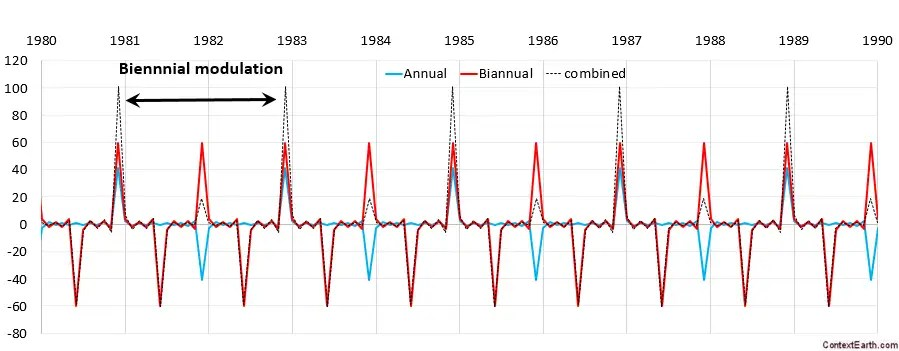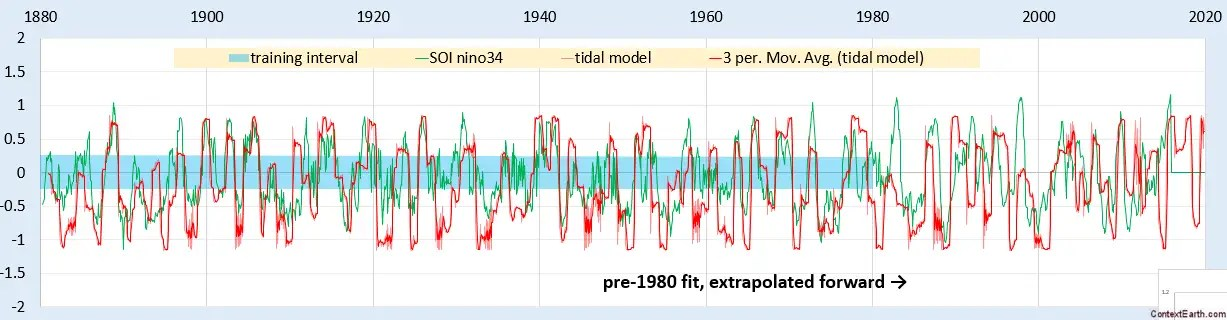# Solution to Pulsed Mathieu Equation

Here is a bit of applied math that I have never seen described before.  It considers solving a variant of the Mathieu differential equation, an unwieldy beast that finds application in models of fluid sloshing (among others). Normally the Mathieu equation is only solvable as a stimulus forcing function convolved against the transcendental Mathieu function. Tools such as Mathematica include the Mathieu function in their library, but any other solution would require a full numerical DiffEq integration.

This is the typical Mathieu equation formulation:$f''(t) + ( omega_0^2 + B cos (nu_0 t) ) f(t) = 0$

But instead of this nonlinear time-varying DiffEq, consider that we replace the sinusoidal modulation with a delta pulse. This is precisely our model of ENSO that we are trying to create from first principles. The pulse represents a forcing impulse from the alignment of a lunar and solar tidal cycle.$f''(t) + ( omega_0^2 + B delta (t) ) f(t) = 0$

Taking the Laplace transform, we quickly arrive at what looks like an ordinary 2nd-order differential equation solution, albeit with an interesting initial condition:$s^2 F(s) + omega_0^2 F(s) + B f(0) = 0$

After taking the inverse Laplace transform, we have:$f(t) = I(t) ast B f(0) delta(t)$

where I(t) represents the impulse response of the first two terms, which is then convolved with a delta function evaluated at a value of f(t) for t=0. This is the only remnant of the nonlinear nature of the classical Mathieu function, in that the initial condition has a scaling proportional to the value of the function at that time. On the other hand, the solution to an ordinary DiffEq would not be dependent on the value of the function.

Thereafter we can extend this to a general solution; by creating a pulse train of delta functions we get this final convolution:$f(t) = I(t) ast B sum_{n=0}^{infty} f(t - n T) delta(t - n T)$

where T is the pulse train period.

This is straightforward to evaluate for any pulse train — all we have to do is keep track of the changing value of f(t) as we come across each pulse.Fig 1: A pulse train with both annual and biannual contributions.

In the above figure, why does the annual pulse have a two-year periodicity? That’s due to the alignment of a non-congruent tidal period with a seasonal pulse in terms of a Fourier series as described here and mathematically refined here.

If that is not intuitive, we can still consider it more of an anzat and see where it takes us.

For an ENSO time series, we an convolve the above delta pulse train with a set of known tidal periods of arbitrary amplitude and phase. For a fit trained up to 1980, this is the extrapolation post-1980:Fig 2: Fit of pulsed Mathieu equation using a set of tidal periods as a forcing function. The impulse response here is a simple year-long rectangular window, i.e. the response has a memory of only a calendar year. This can be further refined if necessary. Yet, the projected time-series evaluated out-of-band from the fitting interval does a good job of capturing the ENSO profile.

The projected waveform matches the last 16 years very effectively considering how noisy the ENSO series is, and is very close to the fit over the entire interval. This is apart from the possible perturbation around the 1982 El Nino. The fact that the monthly tidal periods differ by slight amounts dictates that long multi-decadal intervals should be used for fitting. (In contrast, the model of QBO is primarily Draconic so the fitting interval can be much shorter)Fig 3: Fit over the entire interval. The set of tidal periods applied was limited to the 3 major months (draconic, anomalistic, and sidereal/tropical) and the multiplicative combinations creating the fortnightly tides. This may not be enough to get the details right but erred on the side of under-fitting to establish the physical mechanism.

This approach is the logical follow-on to the wave transformed fitting approach that I had been using, most recently here and here. Both of these approaches are equally clever, which is a necessary ingredient when one is dealing with the unwieldy Mathieu equations. Moreover they both pull out the obvious stationary aspects of the ergodic ENSO time series.

## 2 thoughts on “Solution to Pulsed Mathieu Equation”

1. Pingback: Tidal Model of ENSO | context/Earth